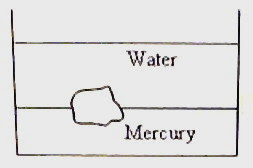## Pages

`“Life is like riding a bicycle.  To keep your balance you must keep moving.”–Albert Einstein`

## Sunday, July 5, 2009

### AP Physics B - Multiple Choice Questions on Fluid Mechanics

Essential things you need to remember in fluid mechanics were discussed in the post dated 3rd December 2007. A few multiple choice questions (with solution) in this section were discussed in the posts dated 4th and 5th December 2007. You can access all posts related to fluid mechanics on this site by clicking on the label ‘fluid mechanics’ below this post.

Today we will discuss a few more multiple choice questions on fluid mechanics:

(1) A water storage tank has a square hole of side 2 cm at its bottom. A plumber, unaware of the hole, admits water into the tank at a constant rate of √2 litre per second. Up to what height can water remain in the tank? (g = 10 ms–2)

(a) 12.25 m

(b) 8.25 m

(c) 2.45 m

(d) 1.25 m

(e) 0.625 m

If h is the height up to which water can remain in the tank, the velocity v of water flowing out through the hole in the steady state is given by

v = √(2gh)

The volume of water flowing out in the steady state is av where a is the area of the hole.

This must be equal to the volume of incoming water which is given as √2 litre = √2 ×10–3

m3. Thus we have

a√(2gh) = √2 ×10–3

Here a = 4 cm2 = 4×10–4 m2.

Substituting, 4×10–4×√(2×10×h) = √2 ×10–3

Or, √(10h) = 2.5 from which h = 6.25/10 = 0.625 m.(2) A piece of granite floats at the interface of mercury and water contained in a beaker (Fig.). If the densities of granite, water and mercury are ρ, ρ1 and ρ2 respectively, the ratio of the volume of granite in water to the volume in mercury is

(a) (ρ2 ρ) /(ρ ρ1)

(b) (ρ2 + ρ) /(ρ+ ρ1)

(c) ρ1 ρ2 /ρ

(d) ρ1 /ρ2

(e) ρ2 /ρ1

The weight of a floating body is equal to the weight of the displaced fluid. If V and v represent the total volume of the piece of granite and volume of granite in water respectively, we have

V ρg = v ρ1g + (V v) ρ2g

Or, v(ρ1 ρ2) = V(ρ ρ2)

Therefore, v/V = (ρ ρ2) /(ρ1 ρ2)

The ratio required in the question is v/ (Vv) and is given by

v/ (Vv) = (ρ ρ2) /[(ρ1 ρ2) (ρ ρ2)]

Or, v/ (Vv) = (ρ ρ2) /(ρ1 ρ) = (ρ2 ρ) /(ρ ρ1)

(3) The pressure of water at the bottom of a water tank exceeds the atmospheric pressure by 104 pascal. The velocity of efflux of water through an orifice at the bottom of the water tank will be (g = 10 ms–2)

(a) √5 ms–1

(b) √10 ms–1

(c) √15 ms–1

(d) √20 ms–1

(e) √30 ms–1

If h is the height of water column in the tank, we have

hρg = 104

Since the density (ρ) of water is 1000 kg m–3,

h×1000×10 = 104 from which h = 1m.

The velocity of efflux (Torricelli’s theorem) is √(2gh) = √(2×10×1) = √20 ms–1

(4) In a wind tunnel the flow speeds (of air) on the upper and lower surfaces of the wing of a model airplane are v1 and v2 respectively (v1> v2). If the wing area is A and the density of air is ρ, the lift on the wing is

(a) ρ (v12 v22)A

(b) ½ ρ (v1 v2)A

(c) ½ ρ (v12 v22)A

(d) ½ (v12 v22)A /ρ

(e) ρ (v1 v2)A

If P1 and P2 are the pressures on the upper and lower surfaces of the wing we have, by Bernoulli’s theorem,

P1 + ½ ρ v12 = P2 + ½ ρ v22

[We have ignored the small height difference between the top and bottom of the wing so that the gravitational potential energy is treated as constant].

The pressure at the top is less than the pressure at the bottom since v1> v2.

The lift on the wing is (P2 P1)A = ½ ρ (v12 v22)A

You will find similar useful multiple choice questions with solution here as well as here at Physicsplus.